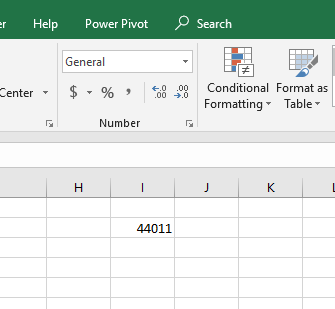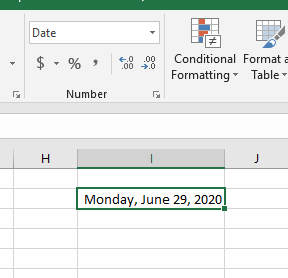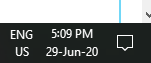PROVIDING ACCURATE, RELIABLE AND STRAIGHT TO THE POINT KNOWLEDGE ABOUT DIFFERENT IT TOOLS EXPLAINED WITH PRACTICAL EXAMPLES

# MANIPULATING DATES AND TIME IN EXCEL

## INTRODUCTION

DATES and TIME , if you are new to Excel and you have already tried using those format, I am pretty sure that you must have felt panicky at some sort of time.
It is quite obvious because when we don’t know the exact working of any system or process, we try to use it just by hit and trial method. It works sometimes and sometimes not.
Here we are talking about Dates and Time in Excel. These are the tricky formats which we need frequently in our reports or charts.
Many times, we need to do operations on them. We need to put them in the conditions to trigger some event which makes it very important for us to learn the exact procedures to perform a task concerned with the dates and time.
In this article we would learn different tricks and methods to handle and manipulate Dates and Time formats so that they don’t mess up with our reports.
• How date works in Excel
• How time is treated by excel
• How to perform many types of frequently used operations directly on the date and time formats.

## HOW DATE IS HANDLED IN EXCEL?

The first and foremost point is to understand  how Excel handles the dates. What are dates for Excel.
Date is treated as a simple serial number by excel starting from Jan 1 1900 [ treated as 1].
From Jan 1, 1900 which is 1 for Excel the serial number starts and it is still going on . For example, it is 29th June 2020 today so the serial number for this date is 44011.
If I type this number in Excel simply,and convert the format to date, It’ll translate it to the date mentioned above which is 29-06-2020.By the pictures shown above it is clear that we can play with the dates in both the ways. We can write the date in the various DATE FORMATS or we can simply use the numbers.[ Of course it is not easy to remember the numbers, but we can refer for once].
EXCEL HAS THE PROVISION OF DATES FROM JAN 1,1900 TO DEC 31, 9999 which corresponds to 2958465.
So it should be clear to the Excel user that date is nothing but a number. But why the problem occurs then.
Problem occurs when we think that the given format is Date but Excel doesn’t accept it as a date. It happens when we violate the rules of entering the date, when we try to enter the date from various formats mentioned below.

## DIFFERENT FORMATS OF DATE RECOGNIZED BY EXCEL

We can enter the date in many formats. Excel gives us the liberty to enter it with numerical or with the words too.
As soon as the date is entered, it recognized and converts the format of the cell to the Date. There are a few mistakes which we do when we enter the date, which doesn’t get converted to DATE and creates problems in our system.
THE FIRST STEP IS TO RECOGNIZE WHICH DATE FORMAT YOU HAVE CHOSE FOR YOUR SYSTEM.
For this, Just take a look at the right bottom of the task bar. [ Right portion of task bar even if taskbar is placed somewhere else].We can see that the format used in the system is DD MM YY. This is very important as only the dates in this format will be recognized as dates by excel otherwise it’ll recognize the dates as TEXT.

FOR DD MM YY SYSTEM, FOLLOWING ARE THE VARIOUS INPUTS AND THEIR RESULTS
 29/6 29 June 2020 29/6/2020 29 June 2020 29 JUNE 2020 29 June 2020 29 JUNE 29 June 2020 29-06-2020 29 June 2020 29-06 29 June 2020 29 JUNE 29 June 2020 29 JUN 29 June 2020 2020-06-29 29 June 2020 JUNE-29-2020 WRONG 29-JUNE-2020 29 June 2020
One of the format , WRONG has been mentioned. You can see that the format tries was the MM DD YYYY and Excel  rejected this format. So it is always important to be careful. Just for the sake of reference , below is the table for OK inputs in MMDDYYYY format.

 29-Jun 29 JUNE 2020 06/29/2020 29 JUNE 2020 JUNE 29,2020 WRONG JUNE 29 29 JUNE 2020 06-29-2020 29 JUNE 2020 06-29 29 JUNE 2020 JUNE 29 29 JUNE 2020 JUN 29 29 JUNE 2020 2020-06-29 29 JUNE 2020 29 JUNE 2020 29 JUNE 2020 JUNE-29-2020 29 JUNE 2020

Few formats which seems to be correct but are not recognized by Excel are

JUNE 29 2020

JUNE 29,2020

JUNE-29/2020

in any of the date system.

## CHANGING THE NUMBER FORMAT TO DATE

One of the very tricky and important format.

EXCEL stores DATE as a serial number counted from JAN 1 1900. And converts this serial number into different types of date formats which we can choose from the given list.

Choose any format from the given list.

Sometimes a problem occurs when we can’t find the format of our choice. Mostly this is due to the location settings. Choose the correct location and you would find your desired format of date.

We can choose the location and EXCEL would populate different formats available from which we can choose our format.

### WHEN SHOULD WE USE DATE NUMBER FORMAT

Whenever we are working with the dates, which is going to be quite often, we should use date format for the ease which is provided by this format while using dates in our calculations.

## HOW TIME IS HANDLED IN EXCEL?

After we have learnt about the DATE HANDLING by Excel, it is time to find out the way TIME is handled. It goes parallel with Date.
As DATE is treated as the serial number, time is taken as the decimal portion of the same serial number.
We know that there are 24*60*60 seconds in a day which comes out to be 86400. So we will divide the decimal part in 86400 portions.
So one second is equal to 1/86400 which comes out to be around 0.00001157 .
One minute is equal to 1/(24*60) which comes out to be around 0.00069444.
BOTH OF THESE CALCULATIONS CAN BE CHECKED MANUALLY TOO.

Now, as we understand that the date is divided into time. It is just like the whole number is divided into the decimal. Let us find out how it works.

It is July 1, 2020 today and its serial number is 44013.
It is 10 AM at my place.
Let us try to find out the fractional part so that Excel interprets it as 10 AM. [We don’t need to do this. It is just for concept building]
The counting starts from 12 AM midnight. Already10 hrs has passed by.
10 hrs =600 minutes.
1 min= 0.00069444
600 minutes= 0.00069444×600=0.416664
Let us type in any cell of excel
44013.416664 and then convert the format of the cell to date and time.

In the picture above, we can see that we put the same fractional number , which we calculated and converted it to the Date and time using the TEXT FUNCTION. The function is visible clearly. The output comes out to be the same from where we had started i.e. July 1 2020 10 AM.

REMEMBER: THE TIME CALCULATION STARTS FROM THE 12:00 AM MIDNIGHT

By this we can find out any time in the day. Some of the standard fractions are .

 TIME FRACTION 12:00 AM 0 6:00 AM 0.25 12:00 PM 0.5 6:00 PM 0.75 10:00 PM 0.916666667

## DIFFERENT FORMATS OF TIME RECOGNIZED BY EXCEL

Just like the DATE , we can enter the time in many USER FRIENDLY formats. Excel accepts most of them.
As soon as we type the time, it recognizes it and converts the format of the cell to TIME FORMAT. But we need to be very careful  about it.
Many times, we think that EXCEL has accepted our value as time, but it hasn’t. We perform the calculations and find wrong results and get confused. So , always be careful about the number formats used in Excel.
Let us have a look at the different values accepted by the Excel easily.
 TIME ENTERED MANUALLY TIME INTERPRETED BY EXCEL 10 AM 10:00:00 AM 10:00 10:00:00 AM 22:00 10:00:00 PM 10:00 PM 10:00:00 PM 10:00:00 10:00:00 AM 10:00:00 AM 10:00:00 AM 10:00:00 am 10:00:00 AM 10:30 10:30:00 AM

These are just for the understanding. These can be easily found in Excel.

#### Steps to find the numerical value of any time in Excel.

• Place the cursor in any cell.
• Enter the time of which you want to find the numerical value.
• As soon as you enter the time, the format of the cell would convert to Custom or Time.
• Go to HOME>NUMBER and change the format to general.
• The time would convert to the fraction.
• That is the fraction for that particular time.

## CHANGING THE NUMBER FORMAT TO TIME

Again very tricky format and very important. The time is stored in excel as the decimal part of the number. For example 0.5 means 12 noon and so on. There are different formats of time from which we can choose how to show the time.

Choose the time format from the given list.

If somehow, can’t find the option of your choice, choose your location and the options will change. Now choose the desired one.

### WHEN SHOULD WE USE TIME NUMBER FORMAT:

We should use time number format when we are working with the time in reports. It is advisable to understand the time format and use it efficiently and it’ll make our job extremely easy.

## INSERT CURRENT DATE AND TIME IN EXCEL

Many times, the current date and time are needed in our applications.

We can insert the current date and time easily.

### STEPS TO INSERT CURRENT DATE IN EXCEL.

•  Select the cell where current date needs to be filled.
• Enter “=TODAY()” [“” need not to be included in formula].
• It’ll give the current date.

### STEPS TO INSERT CURRENT TIME IN EXCEL.

•  Select the cell where current TIME needs to be filled.
• Enter “=NOW()” [“” need not to be included in formula].
• It’ll give the current time including the current date.
• If only time is needed use “=Text(Now(),”hh:mm”)”
• It’ll give only current time.

## FIND THE DATE AFTER FIXED NUMBER OF DAYS

While creating any report , we might come across a situation where we need to find a date after a fixed number of days from a given date.

### STEPS TO FIND THE DATE AFTER A PARTICULAR NUMBER OF DAYS IN EXCEL.

•  Choose the cell where the date after a particular number of days is need to be found.
• Enter the following formula “=cell address of date+number of days after which date is needed”
• As we know date is just a number, we just need to add the number of days and the result will be the date after that many days.

## FIND THE DATE N DAYS BACK

While creating any report , we might come across a situation where we need to find a date  fixed number of days ago from a given date.

### STEPS TO FIND THE DATE N DAYS AGO

•  Choose the cell where the date after a particular number of days is need to be found.
• Enter the following formula “=cell address of date – number of days after which date is needed”
• As we know date is just a number, we just need to subtract the number of days and the result will be the date after that many days.

## COMBINE DATE AND TIME IN EXCEL

Suppose a case where we have date in one cell and time in other cell. If we want to combine them into one cell, follow these steps.

Suppose we have date in one cell and time in other.

### STEPS TO FIND THE DATE N DAYS AGO

•  The solution is very easy. As we know the date is a number and time is the fractional part associated with the same number.
• Enter the following formula in third cell “=cell containing the date+cell containing the time” [“” not included].
• Convert the number format to DATE if needed.

## SEPARATE DATE AND TIME IN EXCEL

Just like we combined the date and time, a need can arise to separate them as well.
Let us take an example where we have a long date i.e. date and time combined. We’ll separate it.
THIS CAN BE SEPARATED WITH THE HELP OF TEXT FUNCTION.
The combined date is present in the cell

### STEPS TO SEPARATE THE DATE AND TIME

•  The combined date and time are put in cell J41. The Date is to be extracted in K41 and TIME in M41.
• Enter the formula in K41 =TEXT(J42,”DD-MM-YYYY”) . It’ll extract the date in the mentioned format i.e. dd mm yyyy.
• Enter the formula for time in M41 as =TEXT(J42,”HH:MM”). It’ll extract the time in the mentioned format i.e. hh:mm. We can also modify it to hh:mm:ss. [Try it yourself]

## BEST WAY TO INSERT A DATE IN EXCEL

If we want to be sure that we face no problems while working with the dates, here is the best way to enter the dates.

Always enter the date using the DATE FUNCTION which is very helpful in handling the dates and we never go wrong with this function.

The syntax of the function is

The Syntax for the function is

=DATE(YEAR, MONTH, DATE)

YEAR PUT THE YEAR IN YYYY FORMAT
MONTH PUT THE MONTH IN MM FORMAT
DATE PUT THE DATE IN DD FORMAT

Now, let us talk about its flexibility.
Even if we put any wrong month or date in this function , it still translates and rectifies the error.
For example.
By mistake we put the date of a JANUARY as 32 it’ll translate it to FEBRUARY 1 rather than giving an error.
Similarly if month goes to 13, it’ll be interpreted as First month of next year.
But yes, we need to always enter the correct date.
DATE FUNCTION HAS THE CAPABILITY TO CONVERT THE TEXT SNIPPETS INTO DATE. WE NEED TO PASS THE TEXT ARGUMENTS IN THE DATE FUNCTION AND IT BECOMES A DATE.
Let us take an example to verify this statement.
Let us put the three values of year, month and date in the cells having the TEXT FORMAT and pass these argument into DATE FUNCTION and check the output.

• ## WHAT IS EXCEL, HOW EXCEL WORKS, WRITE FORMULA IN EXCEL, EXAMPLE OF USING FORMULA IN EXCEL, ANIMATED EXAMLPE OF USING FORMULA IN EXCEL

LEARN

• EXCEL
• JAVASCRIPT
• MORE TO COME…

Encourage us by following at …

If you have any queries or suggestions or any problem which you would like us to solve for you, kindly mail at

[email protected]